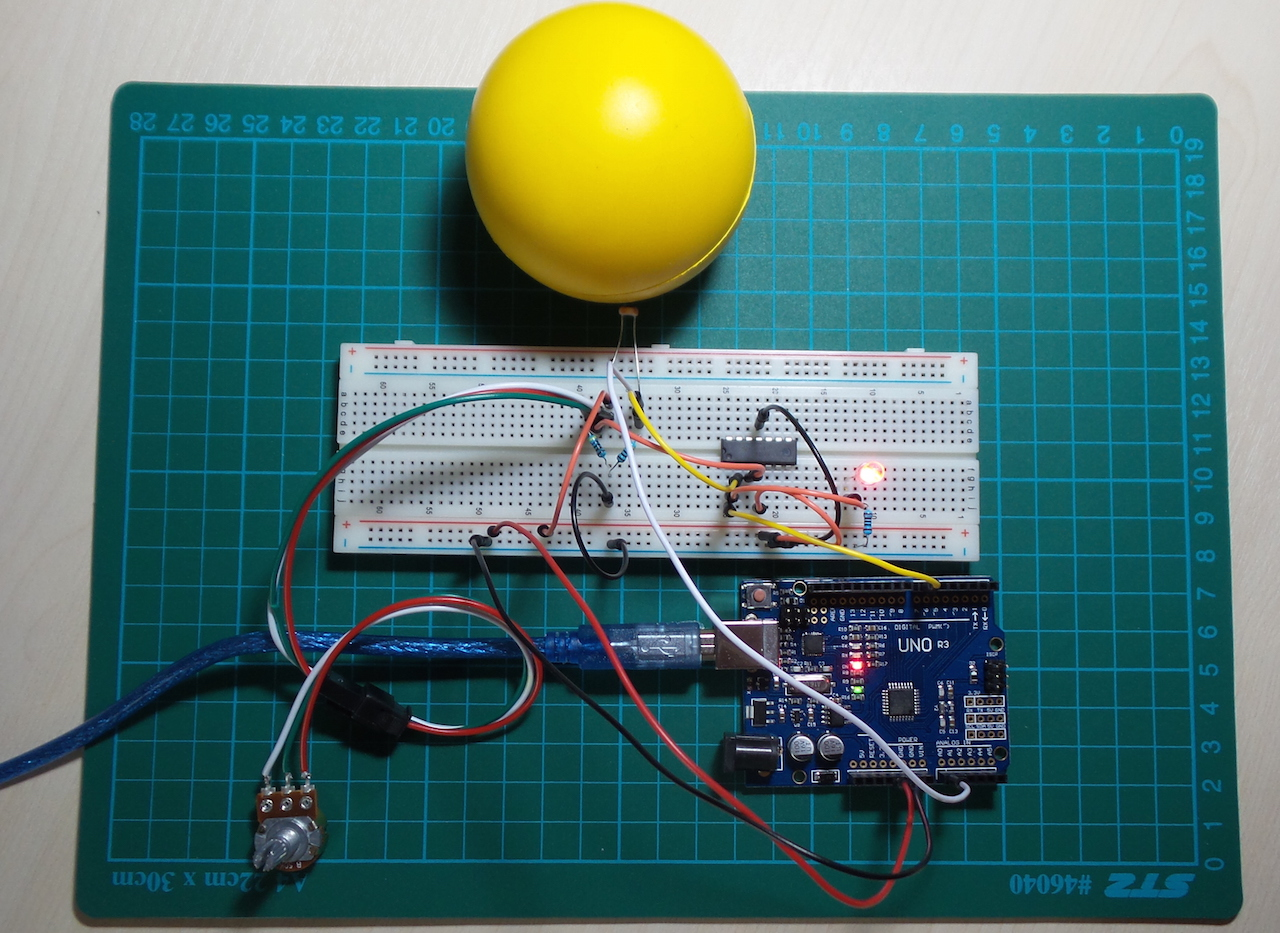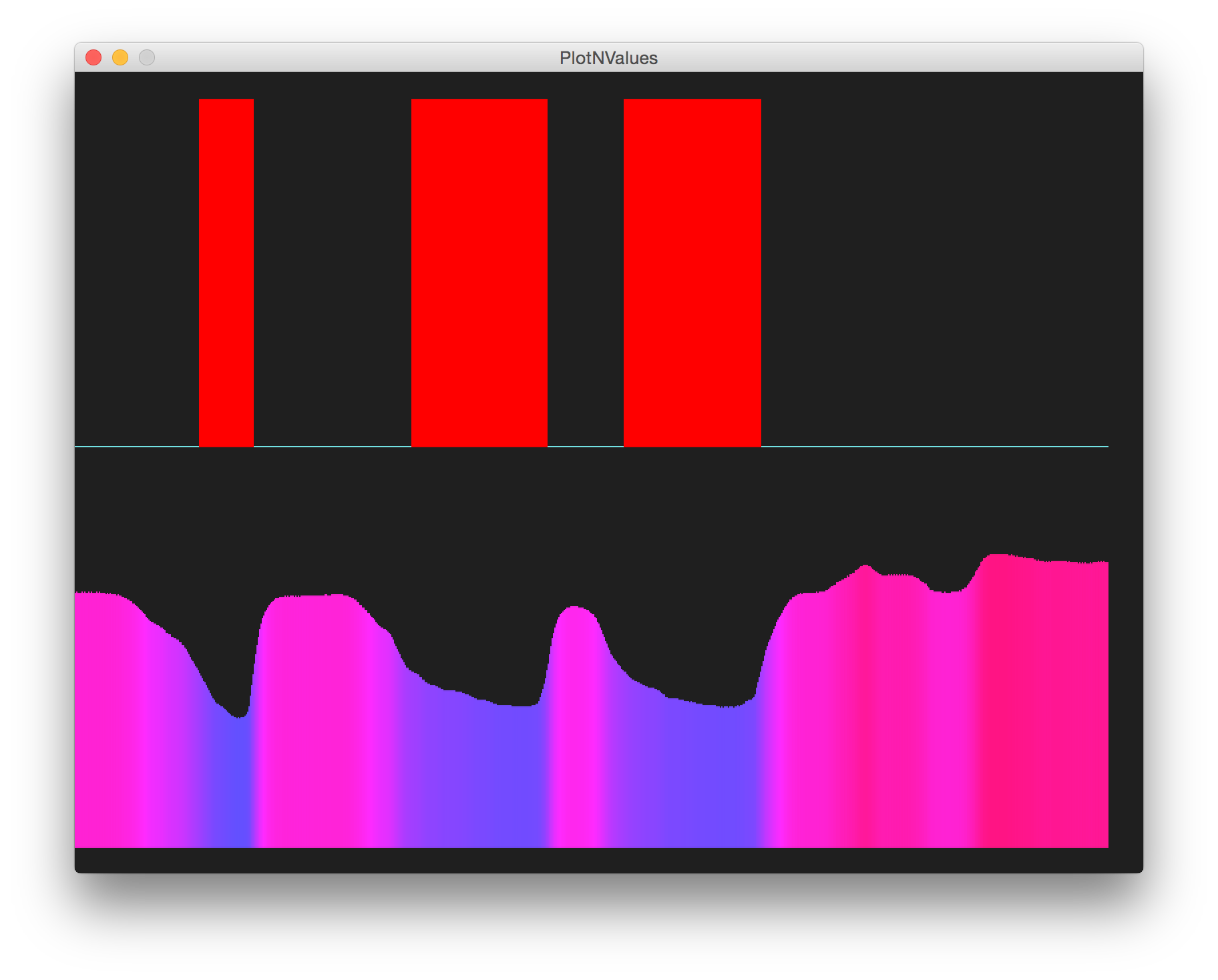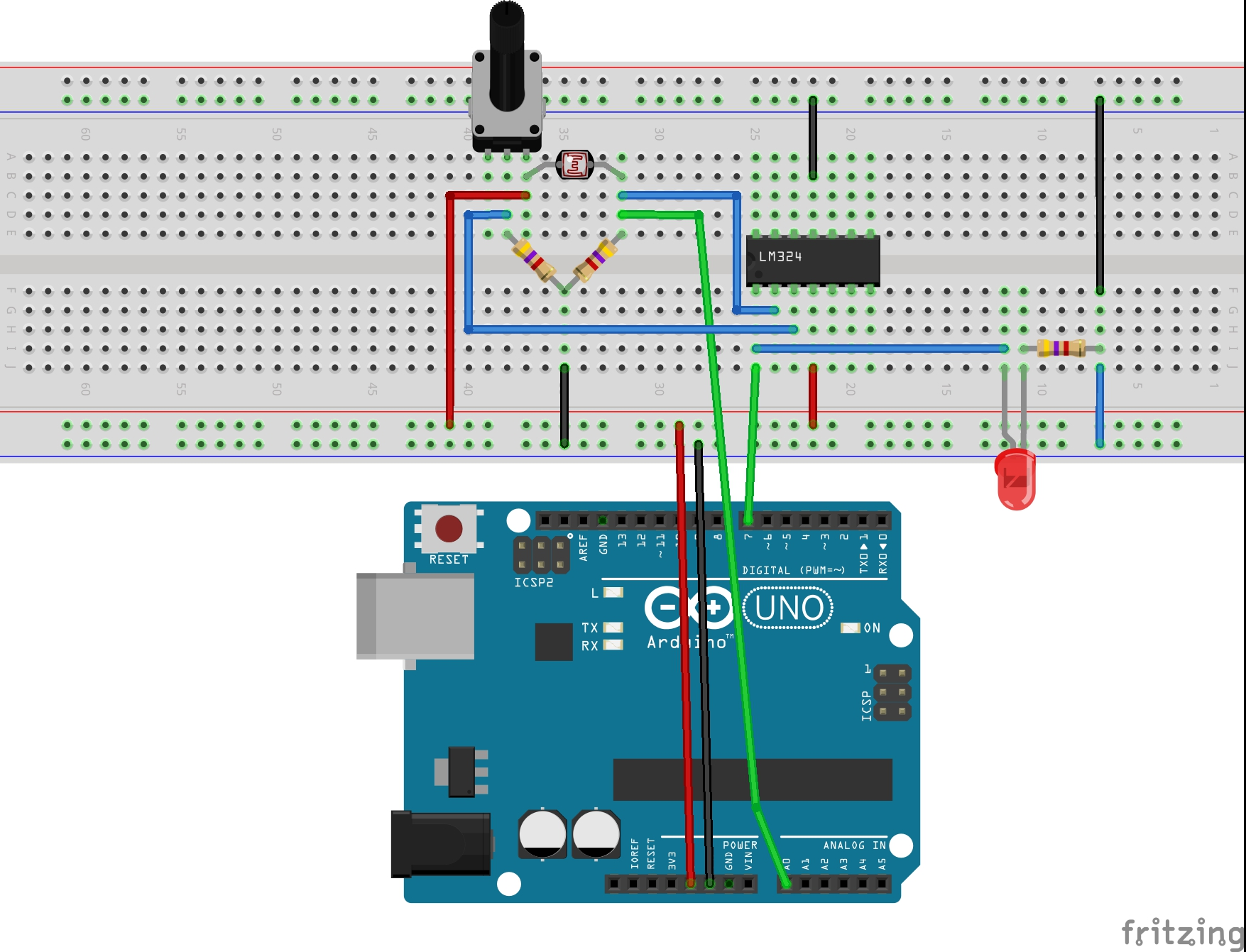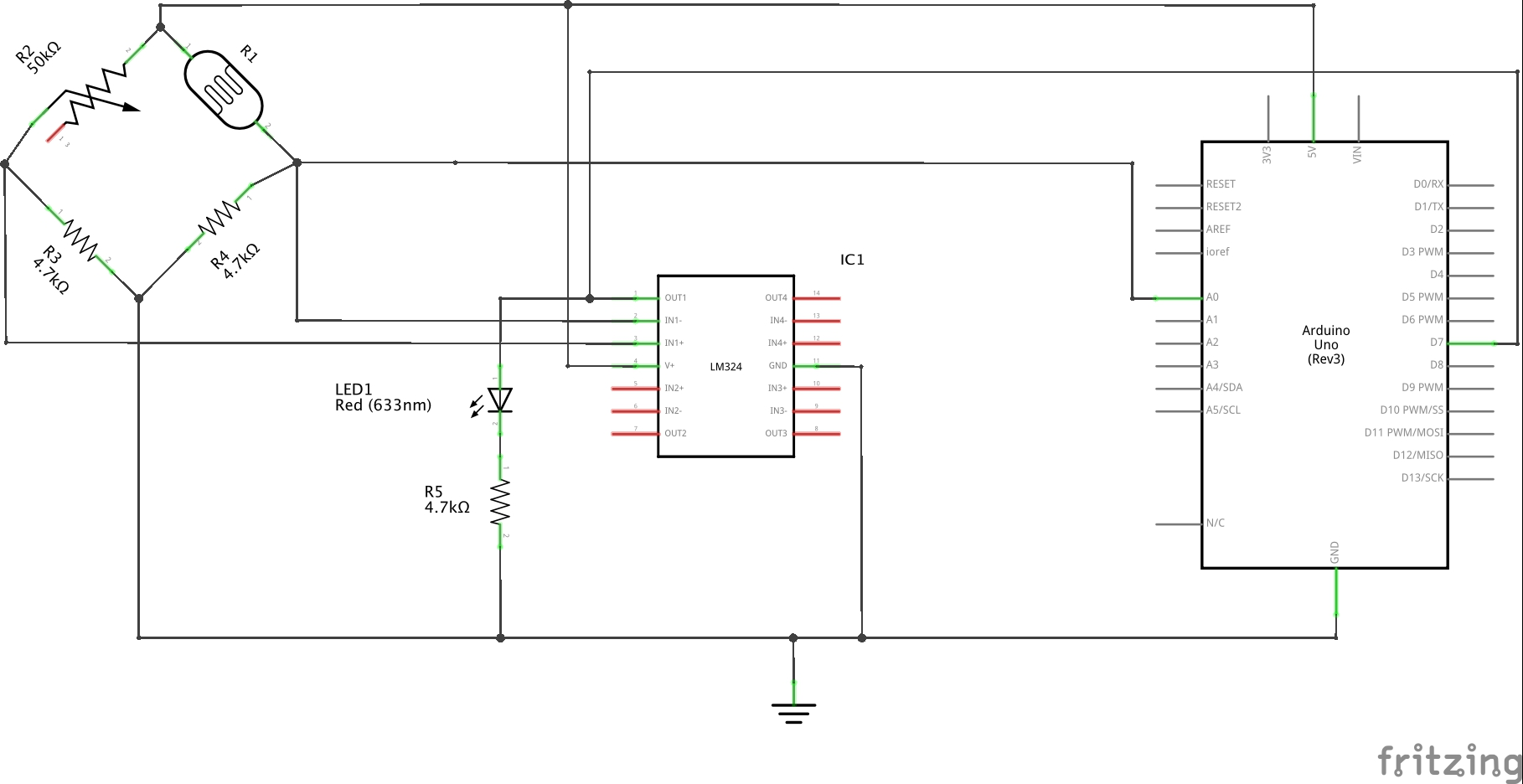# #034 Low-light Trip Detector

Use an Arduino to monitor an LDR sensor, and plot the results with Processing.This demonstrates two circuit concepts:

• a light sensor comprising a light-dependent resistor (LDR) and Wheatstone Bridge.
• a threshold detector using an OpAmp comparator.

The Arduino only acts as a measurement device in this circuit. It reads the voltage at the base of the LDR with an analog input pin, and reads OpAmp comparator status with a digital input pin.

### Calibrating the Wheatstone Bridge

The particular LDR used has a range of about 200Ω (bright light) to 20kΩ (dark). However the ambient light conditions used for testing the circuit exhibited a typical range of 2kΩ-5kΩ, hence 4.7kΩ is selected as the common resistor value in the bridge so that we can drive positive and negative voltages from the bridge. A variable resistor opposes the LDR, so that the actual threshold level can be trimmed.

The voltage differential measured across the bridge - from the base of the pot to the base of the LDR - will tend to rise in lighter conditions as the LDR resistance drops. As conditions get darker, the LDR resistance rises until a point there the voltage differential will go negative.

### Comparator

This voltage differential is fed to an OpAmp comparator (an LM324 Low Power Quad Op-Amp). By feeding the LDR end of the differential to the negative input of the comparator, it acts as a low-threshold trigger. Reversing the inputs to the comparator could be used for a high-threshold trigger.

Note: the circuit as described does not follow best practices for tethering the unused OpAmp units in the LM324 since this is only a test and it didn’t have a noticeable impact on behaviour.

### Behaviour

Here’s a sample trace taken with LEAP#090 PlotNValues (a simple Processing sketch). The lower plot is the voltage at the base of the LDR as I roll a ball in the vicinity of the LDR. The upper plot is the comparator output demonstrating how it flips high when the light level goes below the preset threshold.### Construction